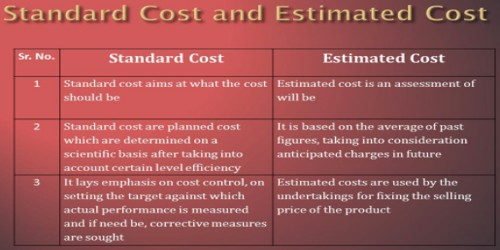Accounting

# Difference between Standard Cost and Estimated CostDifference between Standard Cost and Estimated Cost

Estimated costs are intended to ascertain what the costs will be while standard costs aim at what costs should be. However, both estimated costs and standard costs are related to future period of time but there are some significant differences between them. Some major differences between standard costs and estimated costs are listed below:

• Standard costs, on the other hand, are ascertained on a scientific basis keeping in view certain conditions of efficiency.
• Standard costs are based upon standard rates that are carefully developed and set as scientifically as possible.
• Standard Cost is not generally revised unless it has been set incorrectly or it has become irrelevant to the changed situations.
• Standard costs are used by those organizations that follow standard costing.
• Standard costs are fixed after scientific analysis of relevant cost elements.
• Standard costs are based upon specifications.
• Main purpose of standard costs is to serve as a tool for cost control.
• Standard costs are planned costs which are determined by technical experts after considering levels of efficiency and production

Estimated Cost

• Estimated costs are calculated on the basis of past performance being adjusted in the light of anticipated changes in the future.
• Estimated costs are the expressions of opinion based upon experience.
• Estimated cost is adjusted to the actual cost and expected changes in the coming period.
• Estimated costs are used by those firms that follow historical costing system.
• Estimated costs are based on actual costs and anticipated costs.
• Estimated costs are based on approximation.
• Estimated costs are normally used as guideline for price determination, quoting the selling price etc.
• Estimated costs are determined by taking into consideration the historical data as the basis and adjusting it to future trends.

Information Source: